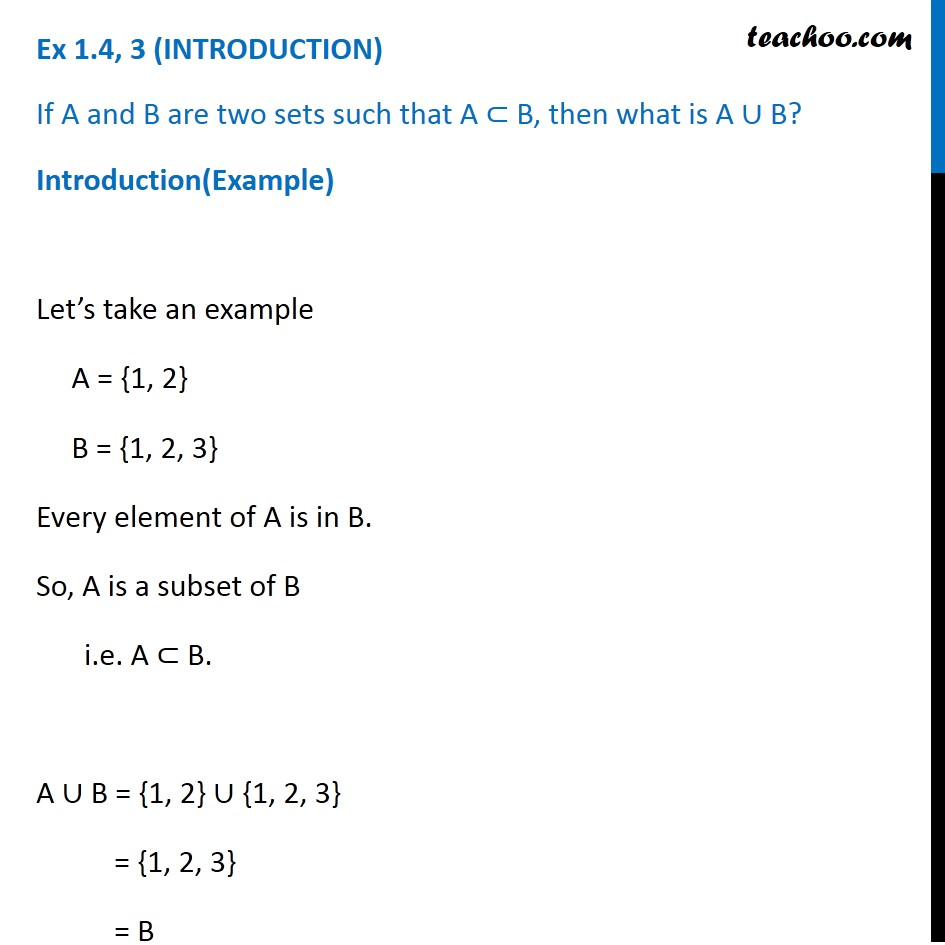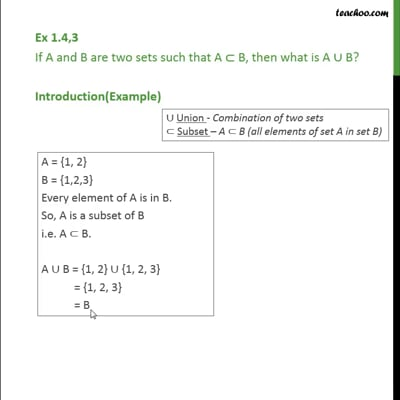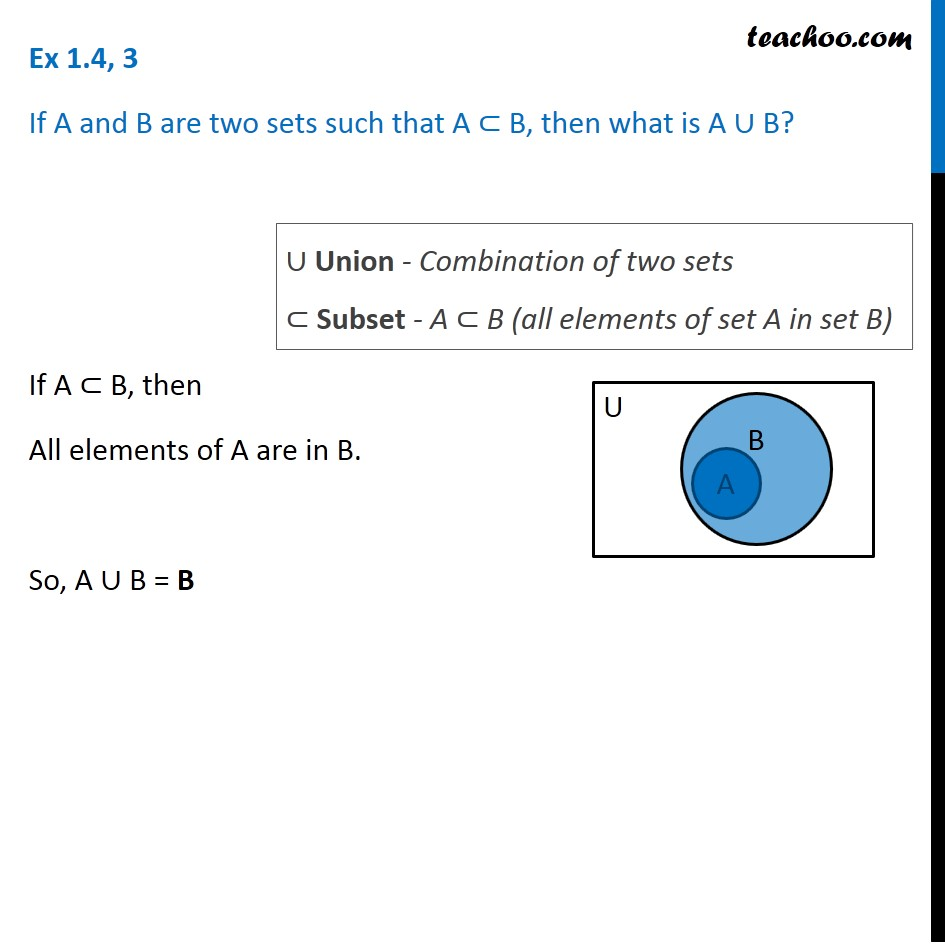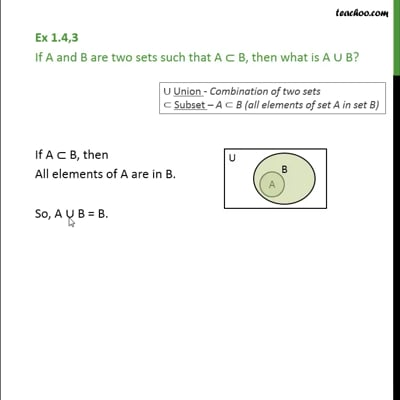Venn Diagram and Union of Set

Chapter 1 Class 11 Sets
Concept wiseThis video is only available for Teachoo black usersThis video is only available for Teachoo black users

Introducing your new favourite teacher - Teachoo Black, at only ₹83 per month

### Transcript

Ex 1.4, 3 (INTRODUCTION) If A and B are two sets such that A ⊂ B, then what is A ∪ B? Introduction(Example) Let’s take an example A = {1, 2} B = {1, 2, 3} Every element of A is in B. So, A is a subset of B i.e. A ⊂ B. A ∪ B = {1, 2} ∪ {1, 2, 3} = {1, 2, 3} = B Ex 1.4, 3 If A and B are two sets such that A ⊂ B, then what is A ∪ B? If A ⊂ B, then All elements of A are in B. So, A ∪ B = B ∪ Union - Combination of two sets ⊂ Subset - A ⊂ B (all elements of set A in set B)